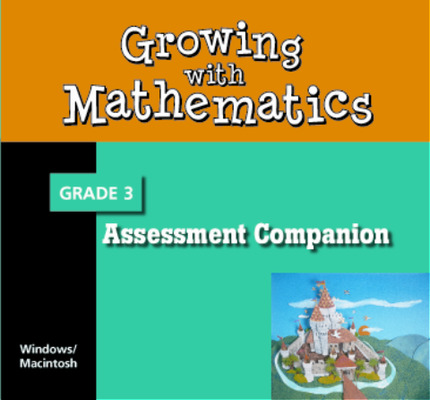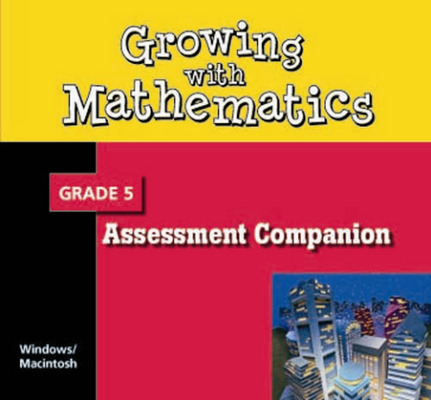Growing with Mathematics

Growing with Mathematics incorporates the best practices from the literature related to teaching young children and mathematics education.Growing With Math's development was informed by the key research that has provided insights into how children learn and the vast amount of information known about the development of mathematical concepts and skills in the elementary grades.

Program Components

•Growing With Math, 100 Number Line Grades 2-4
•Growing with Math, 200 Number Line Grade 3
•Growing with Math, Grade 1, Classroom Kit
•Growing with Math, Grade 1, Discussion Book - Student Hardcover
•Growing with Math, Grade 2, Calendar and Data Kit
•Growing with Math, Grade 2, Classroom Kit
•Growing with Math, Grade 2, Comprehensive Classroom Kit (Includes Classroom Kit and Manipulative Kit)
•Growing with Math, Grade 2, Math Chat
•Growing with Math, Grade 2, Math Chat
•Growing with Math, Grade 3, Classroom Kit
•Growing with Math, Grade 3, Comprehensive Classroom Kit (Includes Classroom Kit and Manipulative Kit)
•Growing with Math, Grade 3, Discussion Book - Student
•Growing with Math, Grade 3, Assessment Companion CD-ROM
•Growing with Math, Grade 5, Assessment Companion CD-ROM
•Growing with Math, Grade 1, Discussion Book - Student
•Growing with Math, Grade 1, Student Book 1
•Growing with Math, Grade 1, Student Book 1
•Growing with Math, Grade 1, Student Book 2
•Growing with Math, Grade 2, Discussion Book - Student Hardcover
•Growing with Math, Grade 2, Discussion Book CD
•Growing with Math, Grade 2, Student Book 1
•Growing with Math, Grade 2, Student Book 1
•Growing with Math, Grade 2, Student Book 2
•Growing with Math, Grade 2, Student Book 2
•Growing with Math, Grade 3, Discussion Book - Student
•Growing with Math, Grade 3, Discussion Book CD
Sort by: